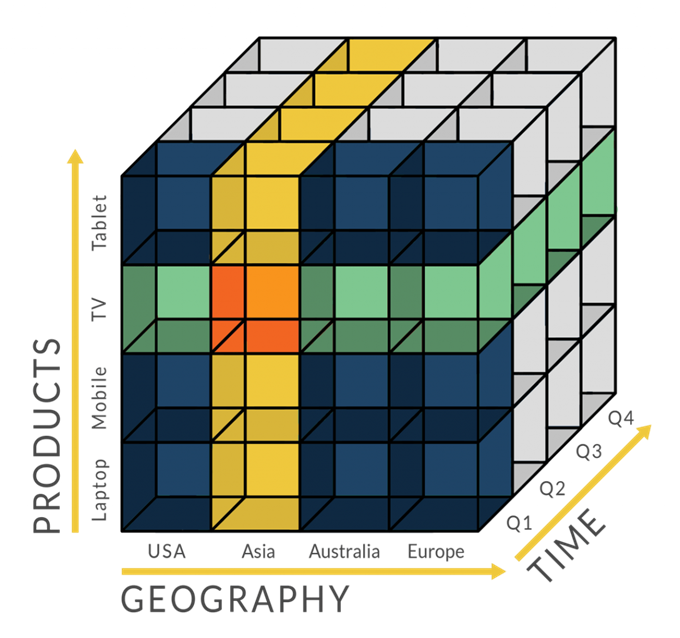# 机器学习算法之主成分分析PCA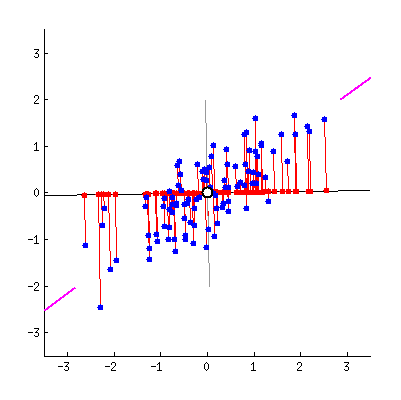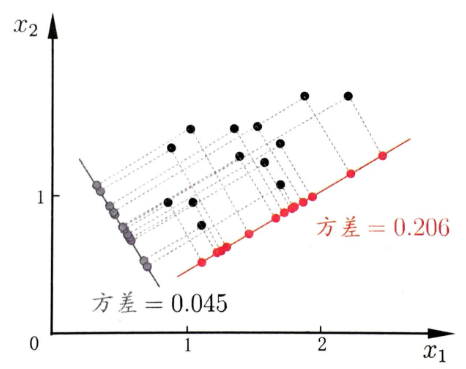## 问题描述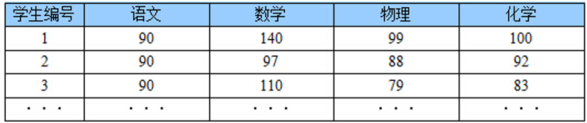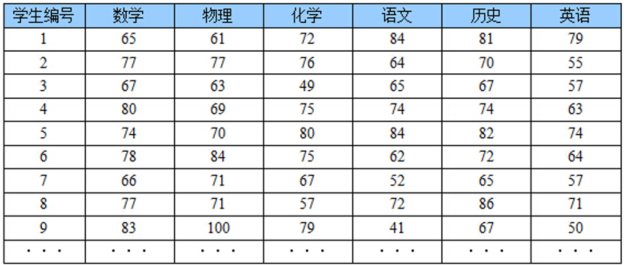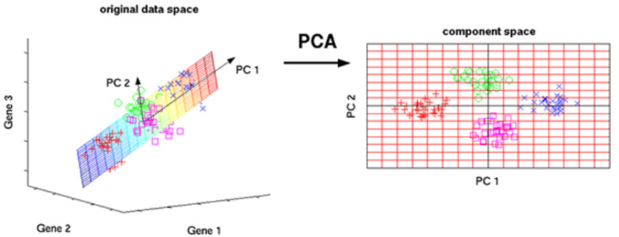## PCA计算实例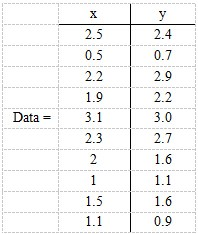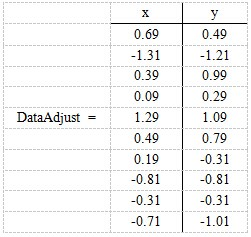$$C = \begin{pmatrix} cov(x,x) & cov(x,y) & cov(x,z)\\ cov(y,x) & cov(y,y) & cov(y,z)\\ cov(z,x) & cov(z,y) & cov(z,z) \end{pmatrix}$$

$$cov = \begin{pmatrix} .616555556 & .615444444\\ .615444444 & .716555556 \end{pmatrix}$$

$$eigenvalue = \begin{pmatrix} .490833989\\ 1.28402771 \end{pmatrix}$$

$$eigenvalue = \begin{pmatrix} -.735178656 & -.677873399\\ .677873399 & -.735178656 \end{pmatrix}$$

FinalData(10*1) = DataAdjust(10*2矩阵) x 特征向量(-0.677873399, -0.735178656)^T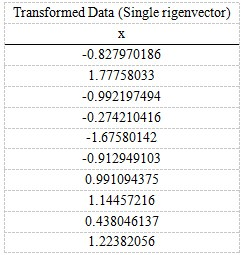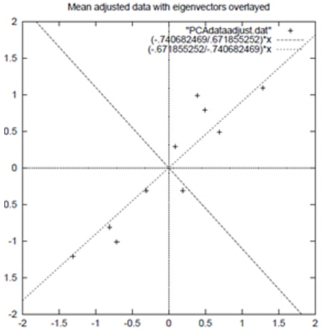## PCA算法原理

$$(a_1,a_2,…,a_n)\cdot (b_1,b_2,…,bn)^T = a_1b_1 + a_2B_2 + … + a_nb_n$$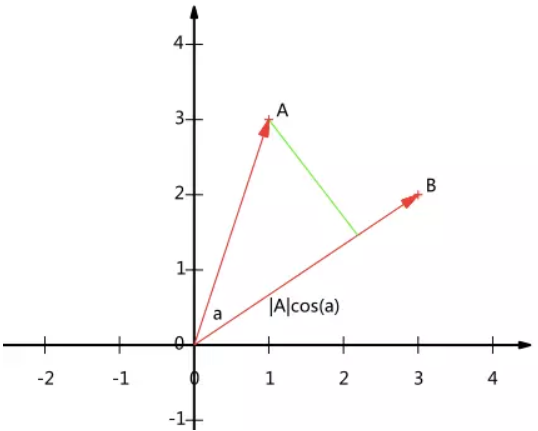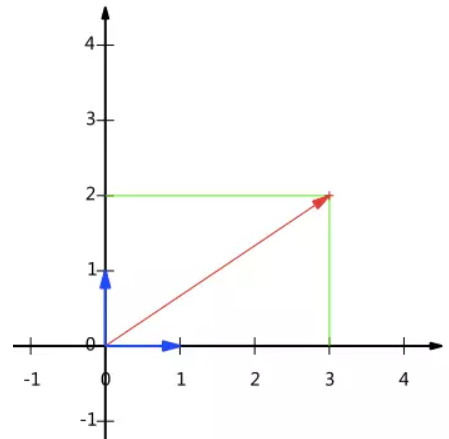$$x(1,0)^\mathsf{T}+y(0,1)^\mathsf{T}$$

$$(\frac{1}{\sqrt{2}},\frac{1}{\sqrt{2}})$$

$$(-\frac{1}{\sqrt{2}},\frac{1}{\sqrt{2}})$$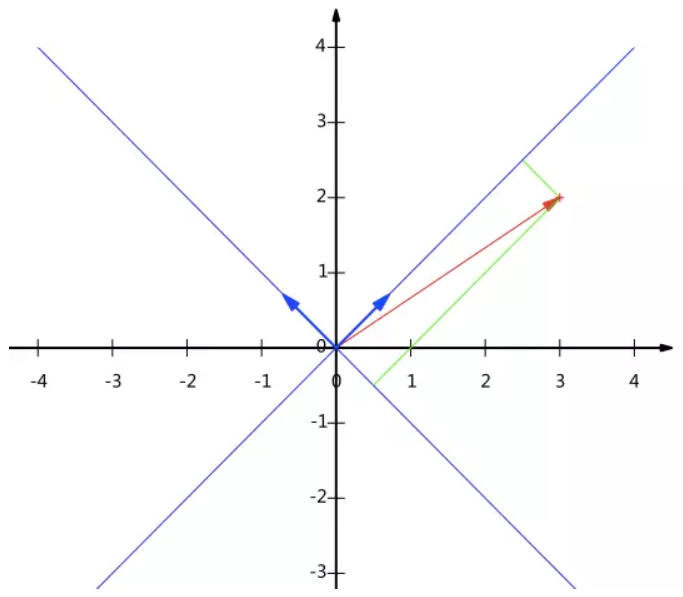$$\begin{pmatrix} 1/\sqrt{2} & 1/\sqrt{2} \\ -1/\sqrt{2} & 1/\sqrt{2} \end{pmatrix} \begin{pmatrix} 3 \\ 2 \end{pmatrix} = \begin{pmatrix} 5/\sqrt{2} \\ -1/\sqrt{2} \end{pmatrix}$$

$$\begin{pmatrix} 1/\sqrt{2} & 1/\sqrt{2} \\ -1/\sqrt{2} & 1/\sqrt{2} \end{pmatrix} \begin{pmatrix} 1 & 2 & 3 \\ 1 & 2 & 3 \end{pmatrix} = \begin{pmatrix} 2/\sqrt{2} & 4/\sqrt{2} & 6/\sqrt{2} \\ 0 & 0 & 0 \end{pmatrix}$$

$$\begin{pmatrix} p_1 & p_2 & \cdots & p_M \end{pmatrix}^\mathsf{T} \begin{pmatrix} a_1 & a_2 & \cdots & a_M \end{pmatrix} = \begin{pmatrix} p_1a_1 & p_1a_2 & \cdots & p_1a_M \\ p_2a_1 & p_2a_2 & \cdots & p_2a_M \\ \vdots & \vdots & \ddots & \vdots \\ p_Ra_1 & p_Ra_2 & \cdots & p_Ra_M \end{pmatrix}$$

$$\begin{pmatrix} 1 & 1 & 2 & 4 & 2 \\ 1 & 3 & 3 & 4 & 4 \end{pmatrix}$$

$$\begin{pmatrix} -1 & -1 & 0 & 2 & 0 \\ -2 & 0 & 0 & 1 & 1 \end{pmatrix}$$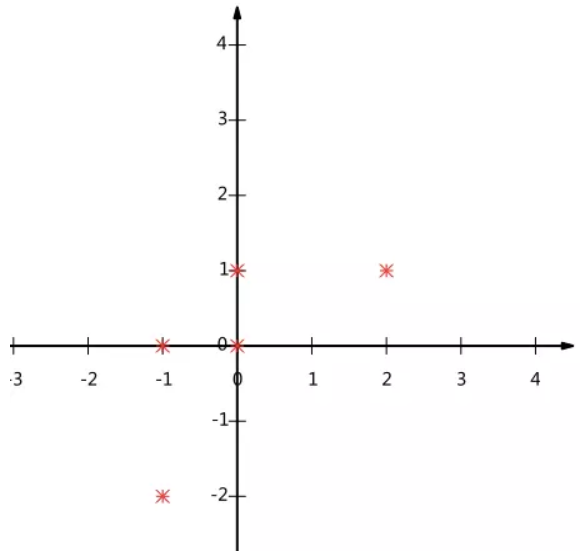$$Var(a) = \frac{1}{m} \sum_{i=1}^m{(a_i-\mu)^2}$$

$$Var(a)=\frac{1}{m}\sum_{i=1}^{m}a_i^2$$

$$Cov(a,b)=\frac{1}{m}\sum_{i=1}^{m}(a_i-\mu )(b_i-\mu )$$

$$Cov(a,b)=\frac{1}{m}\sum_{i=1}^{m}a_ib_i$$

$$X=\begin{pmatrix} a_1 & a_2 & \cdots & a_m \\ b_1 & b_2 & \cdots & b_m \end{pmatrix}$$

$$\frac{1}{m}XX^T = \begin{pmatrix} \frac{1}{m}\sum_{i=1}^{m}a_i^2 & \frac{1}{m}\sum_{i=1}^{m}a_ib_i\\ \frac{1}{m}\sum_{i=1}^{m}a_ib_i & \frac{1}{m}\sum_{i=1}^{m}b_i^2 \end{pmatrix}$$

$$\begin{array}{l l l} D & = & \frac{1}{m}YY^\mathsf{T} \\ & = & \frac{1}{m}(P^TX)(P^TX)^\mathsf{T} \\ & = & \frac{1}{m}P^TXX^\mathsf{T}P \\ & = & P^T(\frac{1}{m}XX^\mathsf{T})P \\ & = & P^TCP \end{array}$$

• 实对称矩阵的特征值都为实数
• 实对称矩阵的所有特征向量正交
• 设特征值重数为r，则必然存在r个线性无关的特征向量对应于，因此可以将这r个特征向量单位正交化

$$E=\begin{pmatrix} e_1 & e_2 & \cdots & e_n \end{pmatrix}$$

$$E^\mathsf{T}CE=\Lambda=\begin{pmatrix} \lambda_1 & & & \\ & \lambda_2 & & \\ & & \ddots & \\ & & & \lambda_n \end{pmatrix}$$

## PCA算法流程

1. 将原始数据按列组成n行m列矩阵X
2. 将X的每一行（代表一个属性字段）进行零均值化，即减去这一行的均值
3. 求出协方差矩阵$C=\frac{1}{m}XX^\mathsf{T}$
4. 求出协方差矩阵的特征值及对应的特征向量
5. 将特征向量按对应特征值大小从左到右按列排列成矩阵，取前k列组成矩阵P
6. $Y=P^TX$即为降维到k维后的数据

import numpy as np

## X: 需要降维的原始数据   topNfeat：需要降到的维数
def PCA(X, topNfeat=9999999):
# 1.原始数据默认都是每一行为一个样本数据，每一列为一个特征，
#   所以进行转置，让每一列代表一个样本数据
X = X.T
# 2.将数据的每一行（代表一个属性字段）进行零均值化
meanValues = np.mean(X, axis=1) #计算每一行的均值
meanValues = np.mat(meanValues).T #将一个向量转换成n*1的矩阵
meanRemoved = X - meanValues   #均值归零

#3.求出协方差矩阵
covMat = np.cov(meanRemoved) #cov计算协方差时除的是 （样本个数-1）,也就是自由度
#     covMat =  meanRemoved @ meanRemoved.T / (meanRemoved.shape)
print("协方差矩阵：\n",covMat)

#4求出协方差矩阵的特征值及对应的特征向量
eigVals, eigVects = np.linalg.eig(covMat)  #eigVals:特征值  eigVects：特征向量
print("特征值\n",eigVals)
print("特征向量\n",eigVects)

#5将特征向量按对应特征值大小从左到右按列排列成矩阵，取前k列组成矩阵
# argsort函数返回的是数组值从小到大的索引值,参数中加个-号，变为从大到小
eigValInd = np.argsort(-eigVals)
eigValInd = eigValInd[0:topNfeat]  #取出前topNfeat个最大的特征值所对应的索引
redEigVects = eigVects[:,eigValInd]  #redEigVects 即为需要的变换矩阵，即P
print("变换矩阵：\n",redEigVects)

#6 Y=P^TX即为降维到k维后的数据
X_PCA = redEigVects.T @ X
return X_PCA

PCA(X, topNfeat = 1)

## PCA算法总结

PCA算法的主要优点有：

• 仅仅需要以方差衡量信息量，不受数据集以外的因素影响。
• 各主成分之间正交，可消除原始数据成分间的相互影响的因素。
• 计算方法简单，主要运算是特征值分解，易于实现。

PCA算法的主要缺点有：

• 主成分各个特征维度的含义具有一定的模糊性，不如原始样本特征的解释性强。
• 方差小的非主成分也可能含有对样本差异的重要信息，因降维丢弃可能对后续数据处理有影响。

## 用scikit-learn进行主成分分析

sklearn.decomposition.PCA参数介绍

PCA类基本不需要调参，一般来说，我们只需要指定我们需要降维到的维度，或者我们希望降维后的主成分的方差和占原始维度所有特征方差和的比例阈值就可以了。现在我们对sklearn.decomposition.PCA的主要参数做一个介绍：

• n_components：这个参数可以帮我们指定希望PCA降维后的特征维度数目。最常用的做法是直接指定降维到的维度数目，此时n_components是一个大于等于1的整数。当然，我们也可以指定主成分的方差和所占的最小比例阈值，让PCA类自己去根据样本特征方差来决定降维到的维度数，此时n_components是一个（0，1]之间的数。当然，我们还可以将参数设置为”mle”, 此时PCA类会用MLE算法根据特征的方差分布情况自己去选择一定数量的主成分特征来降维。我们也可以用默认值，即不输入n_components，此时n_components=min(样本数，特征数)。
• whiten ：判断是否进行白化。所谓白化，就是对降维后的数据的每个特征进行归一化，让方差都为对于PCA降维本身来说，一般不需要白化。如果你PCA降维后有后续的数据处理动作，可以考虑白化。默认值是False，即不进行白化。
• svd_solver：即指定奇异值分解SVD的方法，由于特征分解是奇异值分解SVD的一个特例，一般的PCA库都是基于SVD实现的。有4个可以选择的值：{‘auto’, ‘full’, ‘arpack’, ‘randomized’}。randomized一般适用于数据量大，数据维度多同时主成分数目比例又较低的PCA降维，它使用了一些加快SVD的随机算法。 full则是传统意义上的SVD，使用了scipy库对应的实现。arpack和randomized的适用场景类似，区别是randomized使用的是scikit-learn自己的SVD实现，而arpack直接使用了scipy库的sparse SVD实现。默认是auto，即PCA类会自己去在前面讲到的三种算法里面去权衡，选择一个合适的SVD算法来降维。一般来说，使用默认值就够了。

PCA代码实例

首先我们生成随机数据并可视化：
import numpy as np
import matplotlib.pyplot as plt
from mpl_toolkits.mplot3d import Axes3D
%matplotlib inline
from sklearn.datasets.samples_generator import make_blobs
# X为样本特征，Y为样本簇类别， 共1000个样本，每个样本3个特征，共4个簇
X, y = make_blobs(n_samples=10000, n_features=3, centers=[[3,3, 3], [0,0,0], [1,1,1], [2,2,2]], cluster_std=[0.2, 0.1, 0.2, 0.2],
random_state =9)
fig = plt.figure()
ax = Axes3D(fig, rect=[0, 0, 1, 1], elev=30, azim=20)
plt.scatter(X[:, 0], X[:, 1], X[:, 2],marker='o')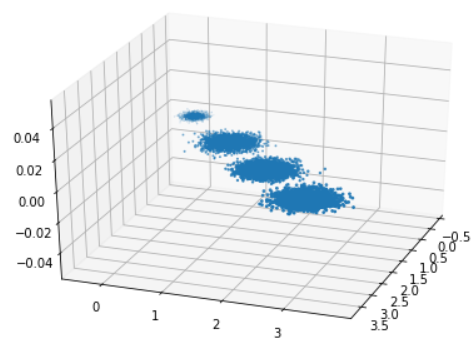from sklearn.decomposition import PCA
pca = PCA(n_components=3)
pca.fit(X)
print(pca.explained_variance_ratio_)
print(pca.explained_variance_)


[ 0.98318212  0.00850037  0.00831751]
[ 3.78483785  0.03272285  0.03201892]


pca = PCA(n_components=2)
pca.fit(X)
print(pca.explained_variance_ratio_)
print(pca.explained_variance_)

[ 0.98318212  0.00850037]
[ 3.78483785  0.03272285]


X_new = pca.transform(X)
plt.scatter(X_new[:, 0], X_new[:, 1],marker='o')
plt.show()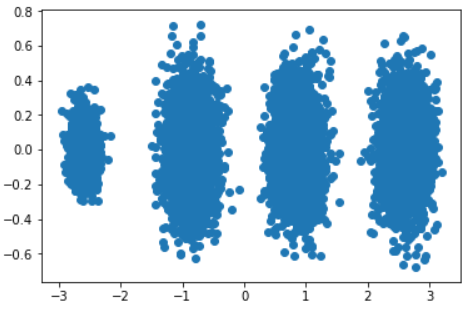pca = PCA(n_components=0.95)
pca.fit(X)
print(pca.explained_variance_ratio_)
print(pca.explained_variance_)
print(pca.n_components_)


[ 0.98318212]
[ 3.78483785]
1


pca = PCA(n_components=0.99)
pca.fit(X)
print(pca.explained_variance_ratio_)
print(pca.explained_variance_)
print(pca.n_components_)


[ 0.98318212  0.00850037]
[ 3.78483785  0.03272285]
2


pca = PCA(n_components= 'mle',svd_solver='full')
pca.fit(X)
print(pca.explained_variance_ratio_)
print(pca.explained_variance_)
print(pca.n_components_)


[ 0.98318212]
[ 3.78483785]
1

TypeError: ‘>=’ not supported between instances of ‘str’ and ‘int’

• KernelPCA类，它主要用于非线性数据的降维，需要用到核技巧。因此在使用的时候需要选择合适的核函数并对核函数的参数进行调参。
• IncrementalPCA类，它主要是为了解决单机内存限制的。有时候我们的样本量可能是上百万，维度可能也是上千，直接去拟合数据可能会让内存爆掉，此时我们可以用IncrementalPCA类来解决这个问题。IncrementalPCA先将数据分成多个batch，然后对每个batch依次递增调用partial_fit函数，这样一步步的得到最终的样本最优降维。
• SparsePCA和MiniBatchSparsePCA。他们和上面讲到的PCA类的区别主要是使用了L1的正则化，这样可以将很多非主要成分的影响度降为0，这样在PCA降维的时候我们仅仅需要对那些相对比较主要的成分进行PCA降维，避免了一些噪声之类的因素对我们PCA降维的影响。SparsePCA和MiniBatchSparsePCA之间的区别则是MiniBatchSparsePCA通过使用一部分样本特征和给定的迭代次数来进行PCA降维，以解决在大样本时特征分解过慢的问题，当然，代价就是PCA降维的精确度可能会降低。使用SparsePCA和MiniBatchSparsePCA需要对L1正则化参数进行调参。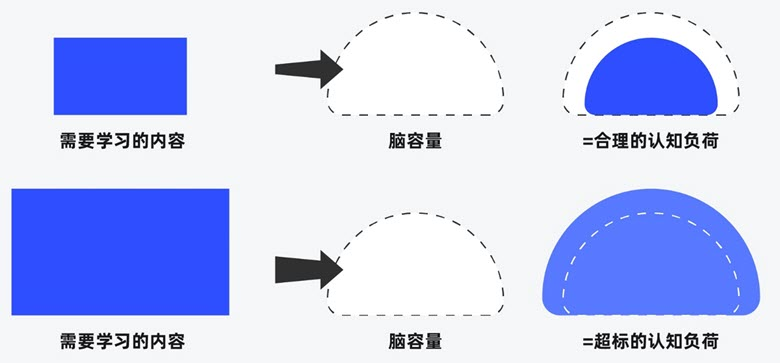##### 界面设计和游戏设计中的认知负荷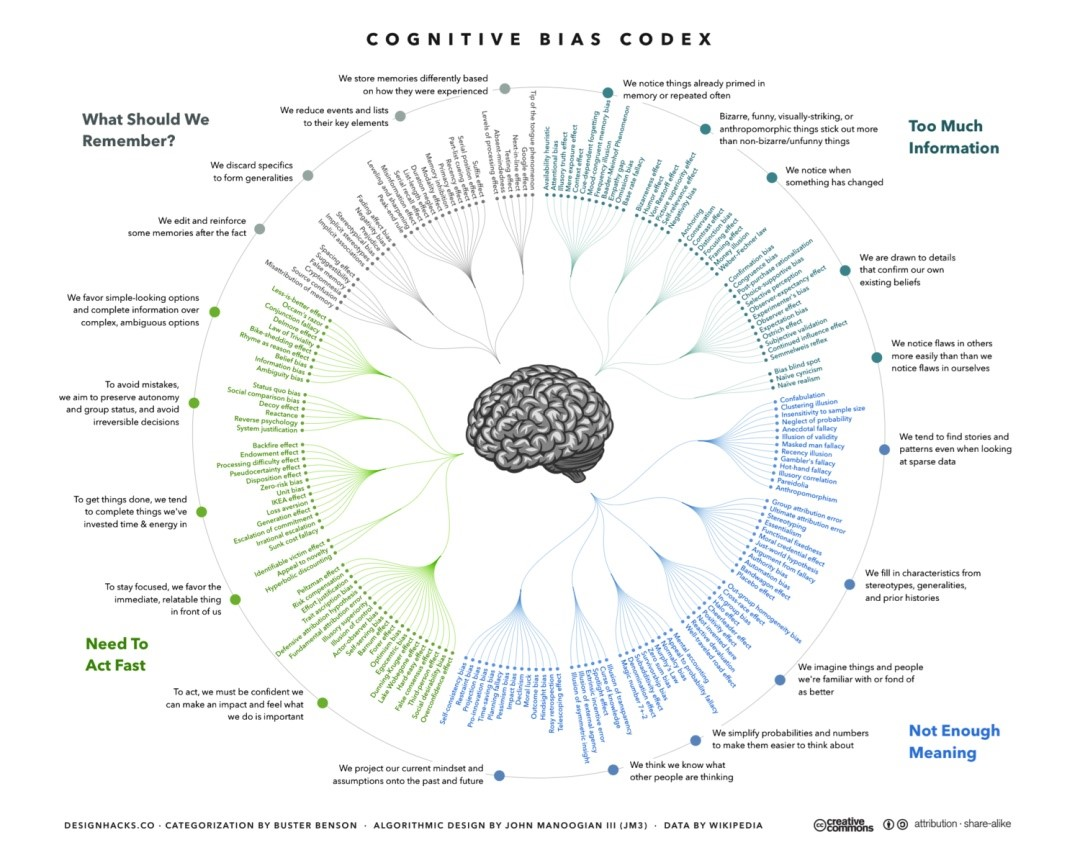##### 理解认知偏差